SCERT AP 7th Class Science Study Material Pdf 5th Lesson Motion and Time Textbook Questions and Answers.

## AP State Syllabus 7th Class Science 5th Lesson Questions and Answers Motion and Time

### 7th Class Science 5th Lesson Motion and Time Textbook Questions and Answers

I. Fill in the blanks.

1. Units of speed is _____
2. If a body covers equal distances in equal intervals of time, it is said to be _____ motion.
3. 60 minutes = _____ seconds. (3600)
4. Average speed = total distance travelled / _____
5. ISRO stands for _____
6. Geostationary satellites are used for _____ purpose.
1. m/s
2. uniform
3. total time taken to travel
4. Indian Space Research Organization
5. Communication

1. The correct relation between speed, distance and time is
a) Speed = distance / time
b) Speed = time / distance
c) Time = speed/ distance
d) Distance = speed / time
a) Speed = distance / time

2. What is common in the following?
Motion of the propeller of a flying helicopter, the hands of a watch, motiob of a giant-wheel.
a) All are examples of translatory motion
b) All are examples of oscillatory motion
c) All are examples of rotatory motion
d) a & c
c) All are examples of rotatory motion

3. Which of the following is not an oscillatory motion?
a) Motion of the hammer of an electric bell.
b) Motion of your hands while running.
c) Motion of a child on a see – saw.
d) Motion of a horse pulling a cart.
d) Motion of a horse pulling a cart.4. Which of the following statements is not correct?
a) The basic unit of time is second.
b) Every object’s motion is Uniform.
c) 1 km/h = 5/18 m/s.
d) Speed is expressed in km/h.
b) Every object’s motion is Uniform.

III. Matching

 A) Rotatory Motion 1. Rocket B) Oscillatory Motion 2. A train entering into a railway station C) Translatory Motion 3. Movement of needle in a sewing machine. D) 100 years 4. Decade E) 10 years 5. Movement of hands in a wrist watch. 6. Century

 A) Rotatory Motion 5. Movement of hands in a wrist watch. B) Oscillatory Motion 3. Movement of needle in a sewing machine. C) Translatory Motion 2. A train entering into a railway station D) 100 years 6. Century E) 10 years 4. Decade

Question 1.
The distance between two stations is 240km. A train takes 4 hrs to cover this distance. Calculate the speed of the train m/s.
The distance between two stations = 240 km
Time taken to cover this distance = 4 hrs
Speed of the train = distance / time
= 240/ 4 km/hrs
= 60 km/hrs

This is the speed of the train in km/hrs,
to convert it into m/s….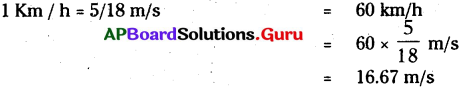Question 2.
Can an object possess translatory and rotatory motion at the same time? Give an example.

1. Yes. An object can possess both translatory and rotatory motions at the same time.
2. When we observe the motion of a cycle tyre moving in a straight line, all the parts of tyre moves in the same direction of cycle so, we can say it is in translatory motion.
3. If we observe the rolling of cycle tyre, it move in a curved (circular) path about a iron rod in the middle of the wheel as an axis. So, we can say it is in rotatory motion.
4. In this way the cycle tyre moving in a straight line possesses both translatory, and rotatory motions.

Question 3.
In a sewing machine used by tailors, mention the type of motion of its parts when it runs, a) the wheel b) the needle c) the cloth

 Parts of sewing machine Type of motion The wheel Rotatory motion The needle Oscillatory or vibratory motion The cloth Translatory motionQuestion 4.
Write the motion of different parts of a bicycle while it is in motion, a) the wheels b) the cycle chains c) the pedal with its arm d) the movement of the rider along with the bicycle.

 Parts of a bicycle Type of motion The wheels Rotatory and translatory motions The cycle chains Translatory motion (curvilinear motion in elliptical path) The pedal with its arm Rotatory motion The movement of the rider along with the bicycle Translatory motion

Question 5.
John tied a stone to a string and whirled it around. What type of motion do you observe?
When a stone is tied to a string and whirled it around, it move in a circular path around the hand. That means the stone fallows a curved path with respect to the hand as a fixed centre or axis of rotation. So it is said to be in rotatory motion.

Question 6.
What are Uniform and Non-Uniform motions? Give four examples for each type of Motions.
i) Uniform motion : “If a body covers equal distances in equal intervals of time, it is said to be in uniform motion”.
Ex: 1. Hands of a clock
2. Revolution of Earth
3. A train going at a steady speed
4. The blades of a fan

ii) Non-uniform motion: If a body covers unequal distances in equal intervals of time, it is said to be in non-uniform motion.
Ex : 1. Movements of butterfly in a garden
2. The motion of a car in a city traffic
3. An aircraft while landing
4. Running of athletes in a marathon

Question 7.
Your friend told you the motion of rocket is fast motion. Will you agree? How can you decide whether the motion of a body is slow or fast?

1. Yes. I agree with him. Because rockets move with the speed of 12 km per second. Its very high speed.
2. The distance travelled by an object in a given interval of time can help us to decide whether it is faster or slower.
3. The distance travelled by an object in a unit time is called as its Speed.
4. Speed = Distance travelled / Time taken to travel.
5. Units of speed is meter per second (m/s) or kilometre per hour (Km/h).
6. 1 Km / h = 5/18 m/s
7. The object moving with less speed is slow and more speed is fast. Thus we can decide whether the motion of a body is slow or fast using its speed.
8. At a particular instant of time, we can find speed of a vehicle using speedometer.

Question 8.
How can you appreciate the clocks and watches in measuring or estimating time?

1. Clocks and watches are helpful to us in measuring or estimating time.
2. They help us in scheduling our works properly.
3. They help us in attending the works on time by displaying the time.
4. With out clocks and watches its very difficult to estimate the time.
5. Sometimes they are used to make a loud alert signal at a specified time to make us wake up or recall a work.
6. Some times they are helpful in measuring the time also.
7. Now a days they are.available in some other gadgets like mobile phone also.
8. Thus they become an essential and integral part of our life.

### 7th Class Science 5th Lesson Motion and Time InText Questions and Answers

7th Class Science Textbook Page No. 68

Question 1.
What do you observe from the picture?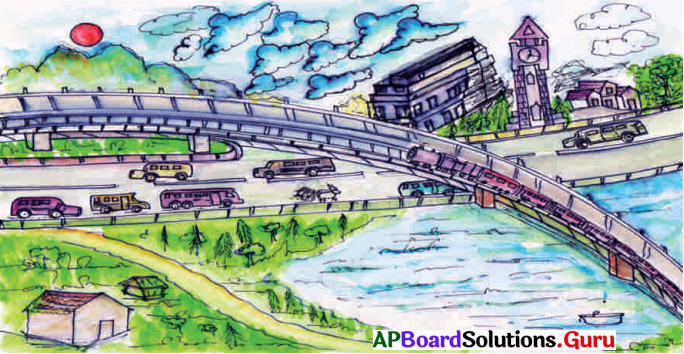You can see the movement of cars, buses, train, boat, clouds, birds and minute hand in the clock etc. On the other side we can see the bridge, buildings, road, hill and the clock tower which are not moving.

7th Class Science Textbook Page No. 69

Question 2.
Do all these things move alike?
No.

Question 3.
What makes the objects move and moving object to come to rest?
Force makes the objects move and moving object to come to rest.

Question 4.
What do we call the motion of a train moving in a straight line?
Translatory motion.Question 5.
Is the forward movement of birds and the movement of its wings same?

1. Not same.
2. Forward movement of birds is a translatory motion and wing movement is an oscillatory motion.

Question 6.
How do you decide whether any object is in motion or at rest?

1. Motion is a common experience in our life.
2. We feel an object is in rest when it is not changing its position and we fee! an object is in motion when it is changing its position with respect to the surroundings.

Question 7.
Are all these trees, buildings, electric poles, etc. really in motion?
No.

Question 8.
Which one do you feel is moving, passenger in the bus or tree?
Passenger.

Question 9.
Which one do you feel is moving, tree or your co-passenger?
Tree.

Question 10.
Why is it so?
Motion and rest are relative terms and ‘hey are relative to the observer. We feel an object is at rest when it is not changing, its position. We feel an object is in motion When it is changing its position with respect to the surroundings.

7th Class Science Textbook Page No. 70

Question 11.
Have you ever played carrom board? What is the reason for movement of coins when we hit them with a striker?
The force applied by the striker on the coins is the reason for movement of coins.Question 12.
How force effects the motion?
Force can change the position of motion to rest, of vice – versa.

7th Class Science Textbook Page No. 71

Question 13.
What did your friend apply to make the ball move?
Force (muscular force).

Question 14.
What did you apply to change the direction of the ball?
Force (muscular force).

Question 15.
What did your friend apply to stop the moving ball?
Force (muscular force).

Question 16.
What can we call the length of path that an object moves from one place to another?
Distance.

Question 17.
Ouserve the picture, Kavitha can reach her school in two ways. From A, B roads which path will you suggest her to reach the school early? You will suggest the shortest distance pat*” which is road A.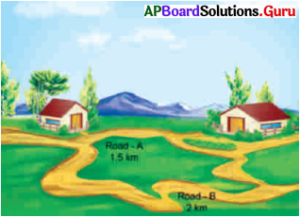7th Class Science Textbook Page No. 72

Question 18.
What can we call the duration of motion of the object when it is moving from one place to another place?
Time to travel

Question 19.
How can we call the period between starting and ending incidents (events) in a running race.
Time.Question 20.
How do we measure or estimate time?
Clocks (watches) are the most common time measuring devices.

7th Class Science Textbook Page No. 73

Question 21.
Are all the motions same?
No, all the motions are not same.

Question 22.
How many types of motions are there?
Motion is of three types :

1. Translatory motion
2. Rotatory motion
3. Oscillatory motion.

Question 23.
Have you played with a toy car? How does it move if you push it forward?
It moves in translatory motion.

Question 24.
Do all the parts of bus like wheels, headlights, windows etc., move through the same distance from building ‘A’ to building ‘B’?
Yes, all parts of the bus moves along the bus.

Question 25.
Is the direction of motion of bus along a straight line or a curved line?
In a straight line.

7th Class Science Textbook Page No. 74

Question 26.
Can you give some more examples of motion in which all points of moving body move through same distance in the same direction?
Examples:

1. Coconut falling from a tree.
2. Movement of lift.
3. Car travelling on a straight road.
4. Movement of water from the tap.

Question 27.
If all the parts of a body move along the curved path. What type of motion it is?
Rotatory motion.

Question 28.
Observe the following diagrams.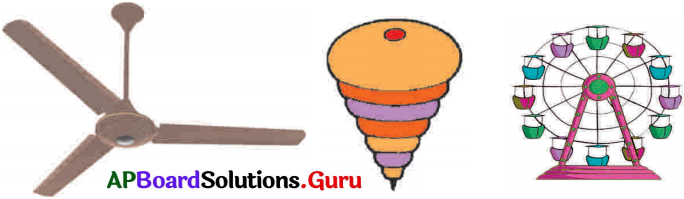1) Do you find any similarity in the above motions?
2) What is the path of motion of each part of the body that moves? ’

1. In the above situations, the parts of the objects move in a curved path with respect to a fixed centre or a fixed axis.
2. They are in rotatory motion with circular path.

7th Class Science Textbook Page No. 75

Question 29.
Can objects have simultaneous Translatory and Rotatory motions?
Yes. The cycle tyre moving in a straight line possesses both translatory and rotatory motions.

Question 30.
Have you played with a see-saw? How does it move?

1. See – saw moves in up and down direction with a fixed point.
2. This type of motion is called oscillatory motion,

Question 31.
How does the needle of swing machine moves?
The needle of a sewing machine moves in ‘to and fro’ motion or back and forth motion.

7th Class Science Textbook Page No. 76

Question 32.
Which similarity do you find in the moving parts of the above instruments?
All are moving in ‘to and fro’ motion.Question 33.
Is the direction of motion constant? Are they moving in the same path again and again?

1. The direction of motion of the objects changes alternatively along the same path about a fixed point. Hence the direction of motion of the object not constant.
2. Yes, they are moving in the same path again and again.

Question 34.
Does all motions take same time to cover same distance?
No.

7th Class Science Textbook Page No. 77

Question 35.
Do you find any relation between distance and time?
Yes, distance divided by time give speed the objects.

Question 36.
How to calculate the average speed?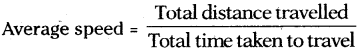Question 37.
Is there any other method to express the motion of objects?
Yes, we can express the motion of objects by graphs.

7th Class Science Textbook Page No. 78

Question 38.
Have you seen launching of rocket in T.V, how does it move?

1. Rockets move in translatory motion.
2. The exhaust gases coming out of the engine’s nozzle at the high speed push the rocket forward.

Question 39.
Do you know which Indian organization is working for it?
ISRO (Indian Space Research Organization) is working for it.

Question 40.
Where do these rockets are launched?
Rockets are launched at Satish Dhawan Space Centre (SDSC – SHAR), Sriharikota, Andhra Pradesh.

7th Class Science Textbook Page No. 80

Question 41.
Have you noticed what happens if you let the air out of a inflated balloon?
The air comes out of balloon in one direction and the balloon moves on the opposite direction with the same speed.

Question 42.
Have you observed the movement of Diwali rocket cracker?
Is there any similarity between movements of rocket cracker and real rocket?

1. Diwali rocket cracker takes flight, when it is fired.
2. Motion of the actual rocket is similar to it.

Question 43.
Have you ever feel wonder of knowing weather report in advance?
Yes, weather report forecasting in advance is possible because of artificial satellites.Question 44.
How google maps give route maps between two places?

1. Google maps is a internet based application to show maps and roots between two places.
2. They map our location with the pictures and data provided by artificial satellites.
3. By using artificial intelligence, they integrate the information and give route maps between two places.

Activities and Projects

Question 1.
Use a tennis ball or cricket to make it translatory motion, rotatory motion, and oscillatory motion. Write a note on it and discuss with your friends.

1. I took a tennis ball and throwed it towards my friend to catch it. The motion showed . by ball is translatory motion.
2. Spun the ball on my finger. It shows rotatory motion.
3. Now it is rolled over the ground towards my friend. It shows both translatory and rotatory motions.
4. Now tied this ball to a thread and moved forward and backward. This shows the oscillatory motion.

Question 2.
Make a water clock or a sand clock and use it to know time.1. I took two, clear plastic bottles that are the same size and shape.
2. Took the caps off, glued them together, and let the glue dry.
3. I Punched a hole through the middle of the glued-together caps.
4. Screwed the cap onto the first bottle, just like we normally would.
5. Filled the second bottle with dry sand.
6. Screwed the empty bottle onto the sand-filled bottle.
7. Tested my sand timer by turning it upside down to know whether the sand is flowing smoothly from one bottle to the next or not.
8. Marked the time on the bottles at various levels of sand by observing the time using stop watch.
9. Wrapped some tape around the neck.
10. My sand clock is ready to use.

Activities

Activity – 1

Question 1.
Classify the objects given in the figure based on motion and rest and what is your inference?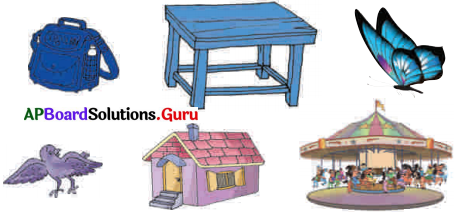Objects at rest Objects in motion

 Objects at rest Objects in motion Bag Butterfly Table Bird House Merry – go-round

Inference:

1. “An object is said to be in motion if it changes its position with respect to its surroundings.”
2. “An object is said to be at rest if there is no change in its position with respect to its surroundings.”

Activity – 2

Question 2.
How do you show that force is required to change the object from rest into motion, to change its direction and even to change from motion to rest?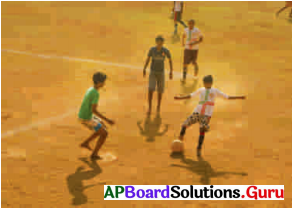Take a football and go to your school ground with your friends. Stand in a triangle shape as shown in the figure.Ask your friend to kick the ball in your direction. You pass the ball to another friend. Ask him to stop that ball. Now answer the following questions.
1) What did your friend apply to make the ball move?
Force

2) What did you apply to change the direction of the ball?
Force

3) What did your Mend apply to stop the moving ball?
Force

You all applied muscular force with your legs to do so.

From the above observation we can say that force is required, to change the object from rest into motion, to change its direction and even to change from motion to rest.Activity – 3

Question 3.
Write any activities to explain the distance and displacement.
Try to measure the distance between your bench to the place where MDM is been served in your school.

How much it is? (Student has to measure and answer on their own)

Have you ever used the google maps to know the distance and direction of your desti-nation?

How much it is? _____ km. (Student has to measure and answer on their own)Observe the picture, Kavitha can reach her school in two ways. From A, B roads which path will you suggest her to reach the school early? You will suggest the shortest distance path which is road A.

From the above activity and examples, we can say that when an object travels from one place to another, the length of the path can be measured and it gives the distance between the two places. When an object travels along the straight-line path from one place to another place, it will reach faster. The length of the straight-line path is the shortest distance between two places, called displacement.

Activity – 4

Question 4.
Conduct 100 meter running race with the help of your physical education teacher. Use stop watch from lab or mobile phone to measure time taken to complete the race by each student. Fill the table given below.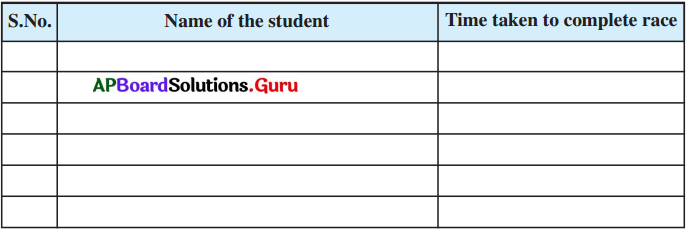Name of the student Time taken to complete race 1. Satyam 1 m 5 sec 2. Venkatesh 53 sec 3. Rama Raju 46 sec 4. Balakrishna 1m 12 sec 5. Narendra 58 sec 6. Vijay 1 min

[Note : Fill in the table with your records)Activity – 5

Question 5.
Consider the following situation. Robert and Kamala measured the distance trav¬elled while coming to school and the time taken to cover that distance. Their data is shown in the Table. You can draw a graph by following the steps given below:

 Time (Minutes) Distance (Meters) 1. 0 0 2. 1 100 3. 2 200 4. 3 300 5. 4 400 6. 5 500

1) Draw two perpendicular lines to represent the two axic anti mark them as OX and OY. Mark OX as time axis and OY as distance axis.

2) Choose a scale to represent distance and time on the graph.
Time : 1 min = 1 cm.
Distance : 50 m = 1 cn

3) Mark values for the time and the distance on the respective axis according to the scale you have chosen.

Now you have to mark the points on the graph paper to represent each set of values and, join al! the points on the graph. This is the distance-time graph for the given motion.

4) If the distance-time graph is a straight line, it indicates that the object is moving with a constant speed. However, if the speed of the object keeps changing, the graph can be of any other shape. From the distance-time graph we can find the speed of object.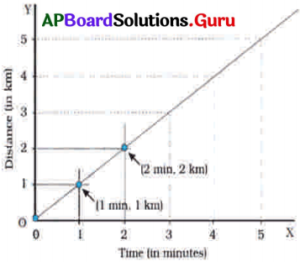From the distance-time graph we can find the speed of object.